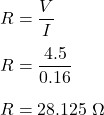## When a light bulb is connected to a 4.5 V battery, a current of 0.16 A passes through the bulb filament. What is the resistance of the filam

Question

When a light bulb is connected to a 4.5 V battery, a current of 0.16 A passes through the bulb filament. What is the resistance of the filament

in progress 0
3 months 2021-07-30T17:35:38+00:00 1 Answers 13 views 0

R = 28.125 ohms

Explanation:

Given that,

The voltage of a bulb, V = 4.5 V

Current, I = 0.16 A

We need to find the resistance of the filament. Using Ohm’s law,

V = IR

Where

R is the resistance of the filament

So,So, the resistance of the filament is equal to 28.125 ohms.